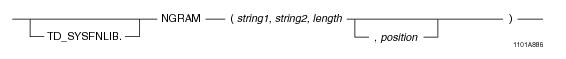# 15.00 - NGRAM - Teradata Database

## Teradata Database SQL Functions, Operators, Expressions, and Predicates

Product
Release Number
15.00
Content Type
Programming Reference
Publication ID
B035-1145-015K
Language
English (United States)

## NGRAM

### Purpose

Returns the number of n-gram matches between string1 and string2.

A high number of matching n-gram patterns implies a high similarity between the two strings.

### Syntaxwhere:

 Syntax element… Specifies… TD_SYSFNLIB the name of the database where the function is located. string1 a character string or string expression. If string1 is NULL, the function returns NULL. string2 a character string or string expression. If string2 is NULL, the function returns NULL. length the value n in n-gram, which is the comparison length. position that the n-gram is a positional n-gram match.

### ANSI Compliance

This is a Teradata extension to the ANSI SQL:2011 standard.

### Invocation

NGRAM is an embedded services system function. For information on activating and invoking embedded services functions, see “Embedded Services System Functions” on page 24.

### Argument Types and Rules

Expressions passed to this function must have the following data types:

• string1 = CHAR, VARCHAR, or CLOB
• string2 = CHAR, VARCHAR, or CLOB
• length = INTEGER
• position = INTEGER
• You can also pass arguments with data types that can be converted to the above types using the implicit data type conversion rules that apply to UDFs.

Note: The UDF implicit type conversion rules are more restrictive than the implicit type conversion rules normally used by Teradata Database. If an argument cannot be converted to the required data type following the UDF implicit conversion rules, it must be explicitly cast.

For details, see “Compatible Types” in SQL External Routine Programming.

### Result Type

If the data type of string1 is CHAR or VARCHAR, the result data type is INTEGER.

If the data type of string1 is CLOB, the result data type is BIGINT.

### Usage Notes

For positional n-gram matching, the position as well as the pattern must match when measuring similarity. The position value indicates how far away positionally the match may be between the 2 strings as follows:

• If position is set to a value of zero, the match must be at the same position in the 2 strings.
• If position is set to a value of x, the match must be within x positions in the 2 strings. For example, if position = 2, then the match must be within 2 positions in the 2 strings.
• As an example, for a string of 'abc', the 1-grams (length =1) are 'a', 'b', and 'c'. The 2-grams (length =2) are 'ab' and 'bc'. The 3-gram (length = 3) is 'abc'. By definition, there are no 4-grams or greater.

The function returns zero in the following cases:

• If the length argument is greater than the length of either string1 or string2.
• If the length argument is <= 0 or if either string1 or string2 is an empty string.
• Patterns beyond the length of 255 are ignored.

### Example

The following query returns a result of 2. The 3-grams 'mit' and 'ith' match. Note that 'Smi' and 'smi' do not match because of the difference in case.

`   SELECT NGRAM('John Smith','Allen smith 1',3); `

### Example

The following query returns a result of zero. There are no 3-grams in the first string expression of '' since the length of the string is less than 3.

`   SELECT NGRAM ('','str1 empty',3); `

### Example

The following query returns a result of zero. There are no 0-grams in the strings.

`   SELECT NGRAM ('test with zero length', 'test with zero length',0); `

### Example

The following query returns a result of 3. The 1-grams 'a', 'b', and 'c' match.

`   SELECT NGRAM ('abc','yyabc',1); `

### Example

The following query returns a result of 2. The 2-grams 'ab' and 'bc' match.

`   SELECT NGRAM ('abc','yyabc',2); `

### Example

The following query returns a result of zero. The 2-grams 'ab' and 'bc' match, but they are not within 1 position of each other.

`   SELECT NGRAM ('abc','yyabc',2, 1); `

### Example

The following query returns a result of 2. The 2-grams 'ab' and 'bc' match, and they are within 2 positions of each other.

`   SELECT NGRAM ('abc','yyabc',2, 2); `

### Example

The following query returns a result of 2. The 2-grams 'ab' and 'bc' match, and they are at the same position in each string.

`   SELECT NGRAM ('abc','abc',2, 0); `

### Example

The following query returns a result of zero. There are no 5-grams since the length of either input string is less than 5.

`   SELECT NGRAM ('abc','abc',5,0); `